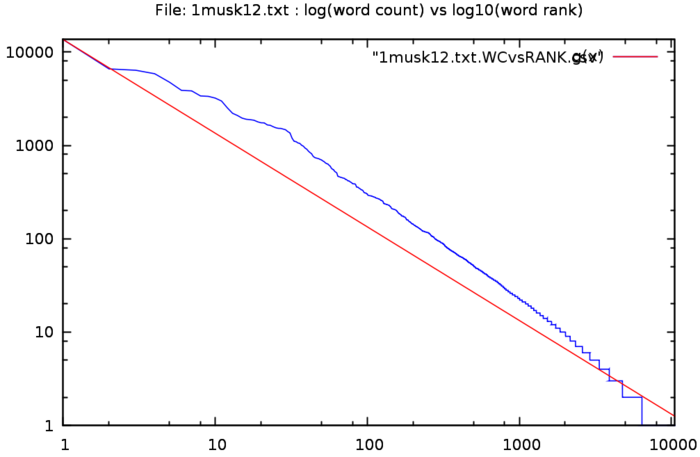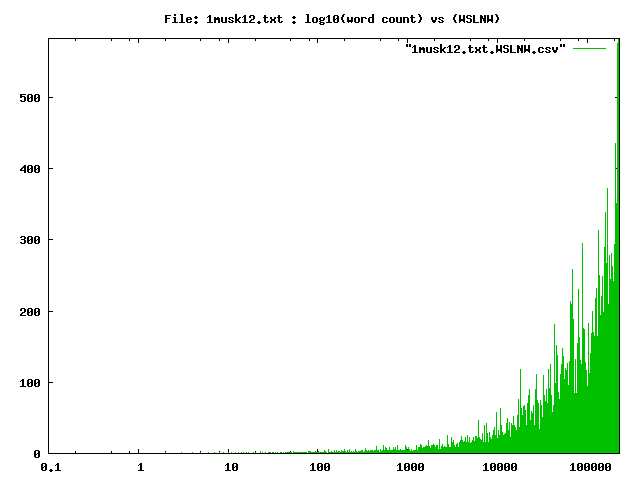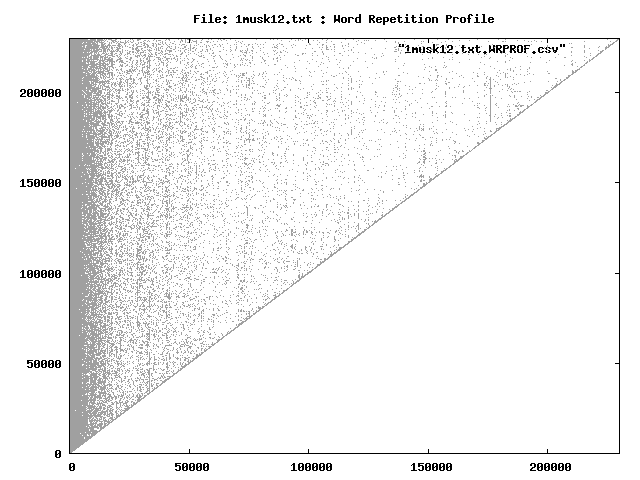Statistics on the text file 1musk12.txt, using the command:zwc -s -d -l -o outfiles -f 1musk12.txt The total word count is 230005 The unique words number 10561 The ratio unique/total is 4.5916 % The highest ranking 59 words make up 50.122 % of the total, OR 0.559 % of the unique words make up 50.122 % of the total volume The number of words used only once 4173 The ratio (words used only once)/total is 1.8143 % The average of the ratios between frequencies is 1.0010